Courses

# Olympiad Test: Analogy - 4

## 20 Questions MCQ Test Science Olympiad Class 6 | Olympiad Test: Analogy - 4

Description
This mock test of Olympiad Test: Analogy - 4 for Class 6 helps you for every Class 6 entrance exam. This contains 20 Multiple Choice Questions for Class 6 Olympiad Test: Analogy - 4 (mcq) to study with solutions a complete question bank. The solved questions answers in this Olympiad Test: Analogy - 4 quiz give you a good mix of easy questions and tough questions. Class 6 students definitely take this Olympiad Test: Analogy - 4 exercise for a better result in the exam. You can find other Olympiad Test: Analogy - 4 extra questions, long questions & short questions for Class 6 on EduRev as well by searching above.
QUESTION: 1

### Directions: Each of the following questions has a group. Find out which one of the given alternatives will be another member of the group or of that class. Q. Pathology, Cardiology, Radiology, Ophthalmology

Solution:

As the all terms given in the question are medical terms and Haematology is also a medical term.

QUESTION: 2

### Directions: Each of the following questions has a group. Find out which one of the given alternatives will be another member of the group or of that class. Q. Wheat, Barley, Rice

Solution:

All the terms given in the question are cereals and gram is also one of the cereals.

QUESTION: 3

### Directions: Each of the following questions has a group. Find out which one of the given alternatives will be another member of the group or of that class. Q. Lock, Shut, Fasten

Solution:

The synonym of Lock, Shut and Fasten is Block.

QUESTION: 4

Directions: Each of the following questions has a group. Find out which one of the given alternatives will be another member of the group or of that class.

Q. Lucknow, Patna, Bhopal, Jaipur

Solution:

All the cities given in the question are state capitals, similarly Shimla is also a capital.

QUESTION: 5

Directions: Each of the following questions has a group. Find out which one of the given alternatives will be another member of the group or of that class.

Q. Tamilian, Gujarati, Punjabi

Solution:

All these words represent the inhabitants of India.

QUESTION: 6

Directions: Each of the following questions has a group. Find out which one of the given alternatives will be another member of the group or of that class.

Q. Clutch, Brake, Horn

Solution:

All these are parts of a vehicle.

QUESTION: 7

Directions: Each of the following questions has a group. Find out which one of the given alternatives will be another member of the group or of that class.

Q. Wrestling, Karate, Boxing

Solution:

These are all defensive games.

QUESTION: 8

Directions: Each of the following questions has a group. Find out which one of the given alternatives will be another member of the group or of that class.

Q. Newspaper, Hoarding, Television

Solution:

All these are used for broadcast.

QUESTION: 9

Directions: Each of the following questions has a group. Find out which one of the given alternatives will be another member of the group or of that class.

Q. Arid, Parched, Droughty

Solution:

The synonym of arid, parched and droughty is dry.

QUESTION: 10

Directions: Each of the following questions has a group. Find out which one of the given alternatives will be another member of the group or of that class.

Q. Engine, Compartment, Wheels

Solution:

All these are related to train.

QUESTION: 11

Directions: In each of the following questions, two sets of figures are given. The first two problem figures bear a certain relationship. Based on the same relationship (analogy), select from answer figures an appropriate figure to replace the question mark in problem figures: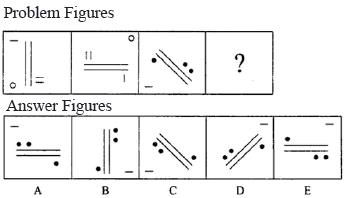Solution:

As per pattern minus sign should be in right corner, parallel lines rotate through 90° and position of dots gets inverted.

QUESTION: 12

Directions: In each of the following questions, two sets of figures are given. The first two problem figures bear a certain relationship. Based on the same relationship (analogy), select from answer figures an appropriate figure to replace the question mark in problem figures: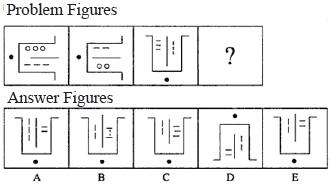Solution:

The inner figures are inverted. The number of lines and dots decrease by one.

QUESTION: 13

Directions: In each of the following questions, two sets of figures are given. The first two problem figures bear a certain relationship. Based on the same relationship (analogy), select from answer figures an appropriate figure to replace the question mark in problem figures: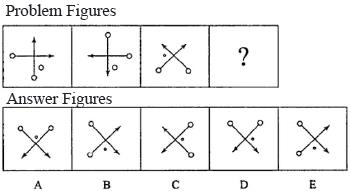Solution:

Two lines are get inverted. The position of dots is changed in order.

QUESTION: 14

Directions: In each of the following questions, two sets of figures are given. The first two problem figures bear a certain relationship. Based on the same relationship (analogy), select from answer figures an appropriate figure to replace the question mark in problem figures: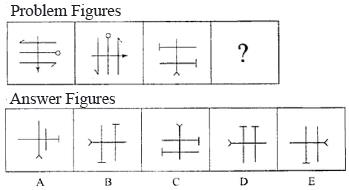Solution:

The figure gets rotated through 90° ACW.

QUESTION: 15

Directions: In each of the following questions, two sets of figures are given. The first two problem figures bear a certain relationship. Based on the same relationship (analogy), select from answer figures an appropriate figure to replace the question mark in problem figures: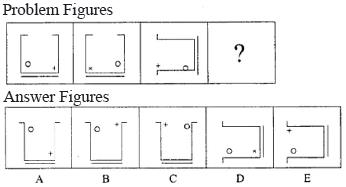Solution:

The inner images are inverted. + sign changes to x.

QUESTION: 16

Directions: In each of the following questions, two sets of figures are given. The first two problem figures bear a certain relationship. Based on the same relationship (analogy), select from answer figures an appropriate figure to replace the question mark in problem figures: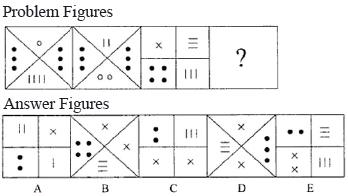Solution:

First and fourth quadrants are same. Second and third quadrants are inverted with two dots decreased and one cross increased respectively.

QUESTION: 17

Directions: In each of the following questions, two sets of figures are given. The first two problem figures bear a certain relationship. Based on the same relationship (analogy), select from answer figures an appropriate figure to replace the question mark in problem figures: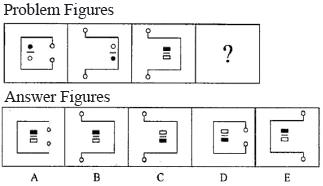Solution:

The figure rotates through 180° and outer side should be inside.

QUESTION: 18

Directions: In each of the following questions, two sets of figures are given. The first two problem figures bear a certain relationship. Based on the same relationship (analogy), select from answer figures an appropriate figure to replace the question mark in problem figures: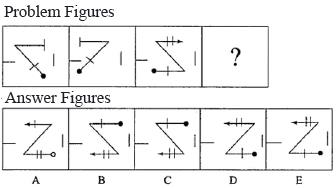Solution:

The figure gets rotated with 180° only, and we get the required image.

QUESTION: 19

Directions: In each of the following questions, two sets of figures are given. The first two problem figures bear a certain relationship. Based on the same relationship (analogy), select from answer figures an appropriate figure to replace the question mark in problem figures: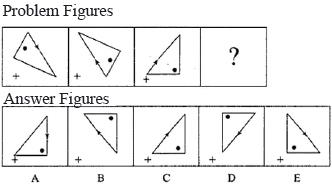Solution:

The figure gets rotated with 180°. The arrow placed on hypotenuse should be in downward direction.

QUESTION: 20

Directions: In each of the following questions, two sets of figures are given. The first two problem figures bear a certain relationship. Based on the same relationship (analogy), select from answer figures an appropriate figure to replace the question mark in problem figures: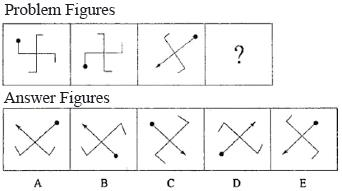Solution:

As per pattern in final image the arrow should be in right corner and in upward direction.